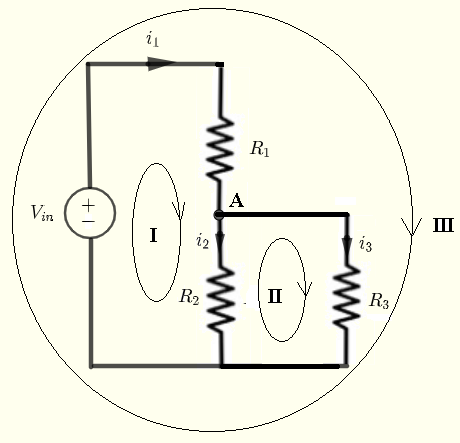# Kirchhoff's Laws Calculator

An online calculator to calculate all the currents and all the voltages in a dc circuit is presented. This calculator may be used to check understanding the use of Kirchhoff's laws of curents and voltages in dc circuits.## Voltages and Currents Formulas

Given $V_{in}$ , $R_1$ , $R_2$ and the load $R_3$, let us find formulas for the currents through and the voltages across all resistors.
Using
Kirchhoff's laws of voltages to write the equations:
$V_{in} = R_1 i_1 + R_2 i_2$    (I)
$- R_2 i_2 + i_3 R_3 = 0$    (II)
$V_{in} = R_1 i_1 + R_3 i_3$    (III)
Using
Kirchhoff's laws of currents at A, we can write the following equation.
$i_1 = i_2 + i_3$    (IV)
Note that we have 4 equations with 3 unknowns: $i_1, i_2$ and $i_3$. If we subtract equations (I) and (II) above, we obtain equation (II). Hence it is sufficient to use two voltage equations only. We use equations (I) and (II) and (IV) to find the three currents.

Use equation (IV) to substitute $i_1$ in equation (I) by $i_2 + i_3$ to obtain two equations with two unknowns $i_2$ and $i_3$
$V_{in} = R_1 (i_2 + i_3) + R_2 i_2$    (I')
$- R_2 i_2 + R_3 i_3 = 0$    (II')
Solve the above system of two equations and two unknowns by any method to obtain
$i_3 = \dfrac{R_2}{R_3(R_1+R_2)+R_1 R_2} V_{in}$
$V_3 = R_3 i_3 = \dfrac{R_2 R_3}{R_3(R_1+R_2)+R_1 R_2} V_{in}$

Currents $i_1$ and $I_2$ are given by
$i_2 = \dfrac{R_3}{R_2} R_3$
$i_1 = i_2 + i_3$
The voltages across $R_1$ and $R_2$ are respectively given by
$V_1 = R_1 i_1$ and $V_2 = R_2 i_2$

The powers delivered to the resistors $R_1$, $R_2$ and $R_3$ are respectively given by
$P_1 = i_1 V_{R_1}$
$P_2 = i_2 V_{R_2}$
$P_3 = i_3 V_3$
The total power $P_t$delivered by the voltage source $V_{in}$ is given by
$P_t = i_1 V_{in}$

## Kirchhoff's Laws Calculator

Enter the resistances of $R_1$ , $R_2$, $R_3$ in Ohms and the source voltage $V_{in}$ in volts as positive real numbers and press "Calculate".

 $R_1$ = 10 $\Omega$       $R_2$ = 20 $\Omega$       $R_3$ = 30 $\Omega$ $V_{in}$ = 12 V Decimal Places = 4